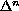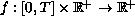Differential Equations and Computational Simulations III
Electron. J. Diff. Eqns., Conf. 01, 1997, pp. 129-136.

### Multiple solutions to a boundary value problem for an n-th order nonlinear difference equation Susan D. Lauer

Abstract:
We seek multiple solutions to the n-th order nonlinear difference equationx(t)= (-1)n-k f(t,x(t)),   t in [0,T]
satisfying the boundary conditions
x(0) = x(1) = ... = x(k - 1) = x(T + k + 1) = ... = x(T+ n) = 0.
Guo's fixed point theorem is applied multiple times to an operator defined on annular regions in a cone. In addition, the hypotheses invoked to obtain multiple solutions to this problem involves the condition
(A)is continuous in x, as well as one of the following:
(B) f is sublinear at 0 and superlinear at infinity, or
(C) f is superlinear at 0 and sublinear at infinity.

Published November 12, 1998.
Mathematics Subject Classifications: 39A10, 34B15.
Key words and phrases: n-th order difference equation, boundary value problem, superlinear, sublinear, fixed point theorem, Green's function, discrete, nonlinear.

Show me the PDF file (118K), TEX file, and other files for this article.

Susan D. Lauer
Department of Mathematics, Tuskegee University
Tuskegee, Alabama 36088 USA